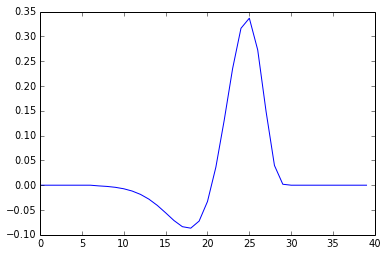In :
%pylab inline

Populating the interactive namespace from numpy and matplotlib

In :
import numpy as np
import matplotlib.pyplot as plt

In :
plt.rcParams['image.cmap'] = 'gray'
plt.rcParams['image.interpolation'] = 'nearest'


The Nipy HRF routines

In :
import nipy.modalities.fmri.hrf as hrfs


Remember tab completion

In :
t = np.arange(0, 25, 0.1)
hrf = hrfs.glovert(t)
plt.plot(t, hrf)
plt.xlabel('time (seconds)')

Out:
<matplotlib.text.Text at 0x1079aedd0>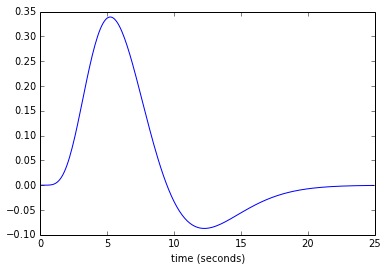What is convolution?

Wikipedia knows: http://en.wikipedia.org/wiki/Convolution

In practice:

In :
dt = 1/8. # time interval in seconds
t = np.arange(0, 40, dt) # times
experiment = np.zeros_like(t)
experiment[t == 5] = 1 # Event at 5 seconds
plt.plot(experiment)

Out:
[<matplotlib.lines.Line2D at 0x1079cce10>]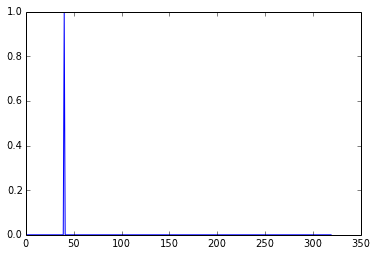We make an HRF at a corresponding time resolution:

In :
hrf_t = np.arange(0, 24, dt)
hrf = hrfs.glovert(hrf_t)


Numpy has a convolve routine:

In :
hrf_experiment = np.convolve(experiment, hrf)
plt.plot(hrf_experiment)
hrf_experiment.shape

Out:
(511,)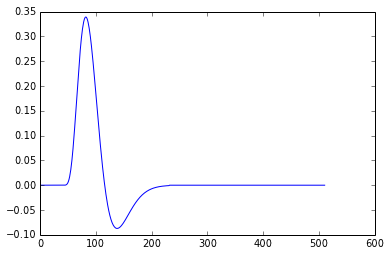What happened? More slowly:

In :
def dumb_convolve(data, kernel):
data_len = len(data)
kernel_len = len(kernel)
out_len = data_len + kernel_len - 1
out = np.zeros((out_len,))
for i, value in enumerate(data):
out[i:i+kernel_len] += value * kernel
return out

In :
plt.plot(dumb_convolve(experiment, hrf))

Out:
[<matplotlib.lines.Line2D at 0x107b19b90>]In :
experiment[t == 15] = 1
plt.plot(dumb_convolve(experiment, hrf))

Out:
[<matplotlib.lines.Line2D at 0x107b39e10>]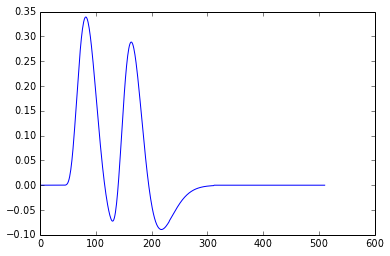Rephrased - the convolution adds a scaled copy of the kernel to the time course, where the scaling comes from the data value at each time point.

In :
block = np.zeros((400,))
block[50:150] = 1
plt.plot(np.convolve(block, hrf))

Out:
[<matplotlib.lines.Line2D at 0x107b6e8d0>]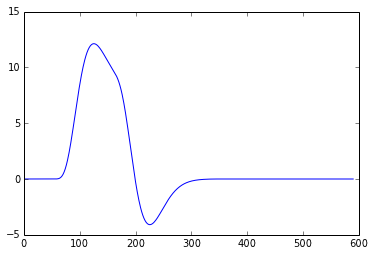Convolution is a little easier to see at coarser detail:

In :
dt = 1. # time interval in seconds
t_low = np.arange(0, 40, 1) # dt == 1
experiment_low = np.zeros_like(t_low)
experiment_low = 1
experiment_low = 1
hrf_low = hrfs.glovert(np.arange(24))
plt.plot(hrf_low)

Out:
[<matplotlib.lines.Line2D at 0x107b9cdd0>]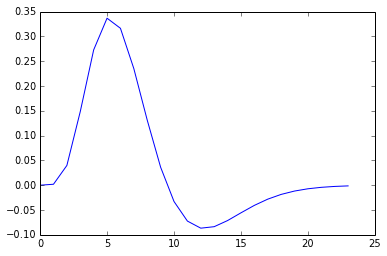Ugly, but, now lets redo the dumb convolution. This time, instead of summing up all the components, (the copies of the HRF corresponding to each time point in the experiment), we'll keep the individual components. There will be one component for each time point in the experiment.

In :
data_len = len(experiment_low)
kernel_len = len(hrf_low)
out_len = data_len + kernel_len - 1
# Let's stack the summed time courses
components = np.zeros((data_len, out_len))
for i, value in enumerate(experiment_low):
components[i, i:i+kernel_len] = value * hrf_low
# Then sum to get the result
out = np.sum(components, axis=0)


This gives the right convolution:

In :
plt.plot(out)

Out:
[<matplotlib.lines.Line2D at 0x107bcbd50>]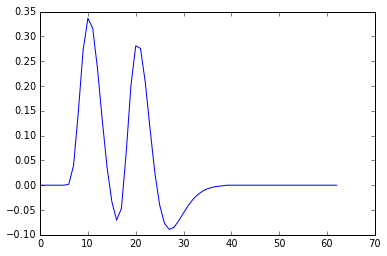In :
plt.imshow(components)
plt.plot((0, data_len), (0, data_len), 'r')
plt.axis('tight')

Out:
(-0.5, 62.5, 40.0, -0.5)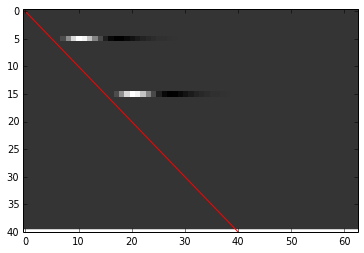## Another way to calculate convolution - using a backwards kernel vector¶

Looking at the components matrix give us another way to calculate the convolution.

Notice that each component starts at the red line. The sums to get the convolution result are over the columns.

What is this sum made up of? First we need some notation.

Call our data vector $d$ ($d$ is experiment_low in the case above). $d$ has $D$ elements $d_1, d_2 ... d_D$ ($D$ is data_len above). Call our kernel vector $k$ ($k$ is hrf_low above). $k$ has $K$ elements $k_1, k_2, ... k_K$ ($K$ is kernel_len above).

Let's call $\mathbf{C}$ the $D$ x $M$ matrix of $D$ components (rows) and $M = D+K-1$ out values (columns). $\mathbf{C}[i, j]$ is the value in row $i$ and column $j$.

Consider the sum of column $j$. If $v$ is the output vector from the convolution, $v_j$ is the sum of column $j$ of $\mathbf{C}$.

All values of $\mathbf{C}[i>j, j]$ are zero because the components start at diagonal cells $\mathbf{C}[i, j]$ where $i = j$.

At the diagonal we have the data value at this time point times the first value in the kernel: $\mathbf{C}[j, j] = d_j * k_1$.

Working upwards from the diagonal, $\mathbf{C}[j-1, j]$ is the second element of the vector resulting from multiplying the kernel with data value $d_{j-1}$ so $\mathbf{C}[j-1, j] = d_{j-1} * k_2$. The kernel goes to $0$ after $K$, so the whole column sum is given by:

$$\sum_{i=1}^M{C(i, j)} = d_j * k_1 + d_{j-1} * k_2 + ... + d_{j-(K-1)} * k_K$$

(if we run out of data ($d_i$ where $i < 1$ or $i > D$) assume the value is zero).

This formula gives another way to do the convolution. We step through each value in the data, and starting at that point, go back through the data and the kernel, summing up the data times the kernel values. This is the direct implementation of the column sum formula above. Like this:

In :
def ugly_convolve(data, kernel):
# Starts the same as the dumb convolve
data_len = len(data)
kernel_len = len(kernel)
out_len = data_len + kernel_len - 1
out = np.zeros((out_len,))
# Different here though
for i in range(out_len):
# Sum up the value of the data * kernel going backwards through the data
val = 0
# Go back over the kernel and data
for k in range(kernel_len):
# Go back from current point in data
data_ind = i - k
# If we've run out of data, assume 0
if data_ind < 0 or data_ind > data_len - 1:
d_val = 0
else:
d_val = data[data_ind]
# Multiply the corresponding kernel value with data
val = val + d_val * kernel[k]
out[i] = val
return out

In :
ugly_out = ugly_convolve(experiment_low, hrf_low)
plt.plot(ugly_out)
assert np.all(ugly_out == out)## Another way to think of convolution - filter matrices¶

We can also do convolution with a filter matrix using the elements of the column sum formula. Here it is again:

$$\sum_{i=1}^M{C(i, j)} = d_j * k_1 + d_{j-1} * k_2 + ... + d_{j-(K-1)} * k_K$$

We can split this formula into the data part and the kernel part. We'll make a matrix representing the kernel part by constructing a matrix with the $k_1, k_2 .. k_K$ values going upwards along the columns from the diagonal, for each column. For our HRF kernel, we could call this the upward HRF matrix.

Then we'll make a matrix for the data part of the formula; the $d_j, d_{j-1} ...$ from the equation above.

Multiplying the elements of the matrix for the kernel part and the matrix for the data part reconstructs the components matrix above.

We first make the upwards HRFs like this:

In :
up_hrfs_rows = kernel_len - 1 + data_len + kernel_len - 1
up_hrfs = np.zeros((up_hrfs_rows, out_len))
backwards_hrf = hrf_low[::-1]
for i in range(0, kernel_len -1 + data_len):
up_hrfs[i:i+kernel_len, i] = backwards_hrf
plt.imshow(up_hrfs)

Out:
<matplotlib.image.AxesImage at 0x1082fb3d0>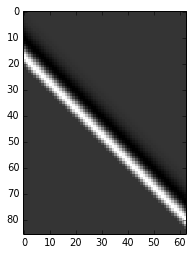We can chop off the top of and bottom of these upwards HRFs because they'll be multiplying outside the data range. Once we've done this we've got something the same shape as components above:

In :
up_hrfs_trimmed = up_hrfs[kernel_len-1:kernel_len-1+data_len, :]
plt.imshow(up_hrfs_trimmed)
plt.plot((0, data_len), (0, data_len), 'r')
plt.axis('tight')
up_hrfs_trimmed.shape

Out:
(40, 63)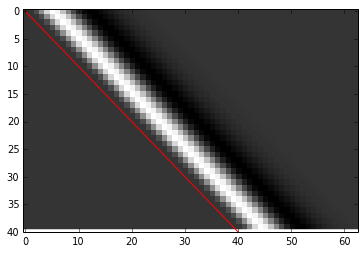Think of each column as being an HRF flipped to go upwards rather than downwards. For example, here are columns 28 through 31:

In :
fig, axes = plt.subplots(4, 1)
for i in range(4):
axes[i].plot(up_hrfs_trimmed[:, 28+i])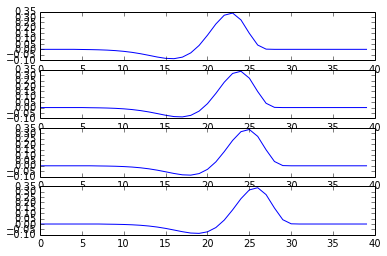We can make another matrix to give the data corresponding to each upwards HRF. These are the $d_j, d_{j-1} ...$ values from the column formala above. Of course the $d$ values are just the $d$ vector repeated for each column.

In :
data = np.tile(experiment_low[:, None], (1, out_len))
plt.imshow(data)

Out:
<matplotlib.image.AxesImage at 0x107bfec10>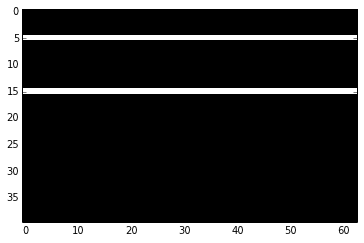If we do an element-wise multiply the of the data matrix with the upward HRFs, we get the components back:

In :
plt.imshow(data * up_hrfs_trimmed)
plt.plot((0, data_len), (0, data_len), 'r')
plt.axis('tight')
assert np.all(data * up_hrfs_trimmed == components)In :
upwards_out = np.sum(data * up_hrfs_trimmed, 0)
assert np.all(out == upwards_out)


We can do the same thing by taking the transposed upwards HRF matrix and matrix multiplying by the data vector. The transposed upwards HRF matrix is now called a filter matrix because the matrix multiplication applies the HRF convolution 'filter' to the data:

In :
filter_matrix = up_hrfs_trimmed.T
plt.imshow(filter_matrix)
filtered_out = filter_matrix.dot(experiment_low)
assert np.all(filtered_out == out)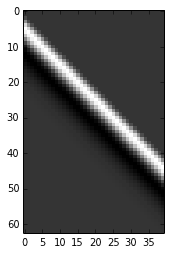In the transposed (filter) matrix, the HRFs are now going backwards instead of upwards:

In :
plt.plot(filter_matrix[30, :])

Out:
[<matplotlib.lines.Line2D at 0x109a2f450>]